### Choose language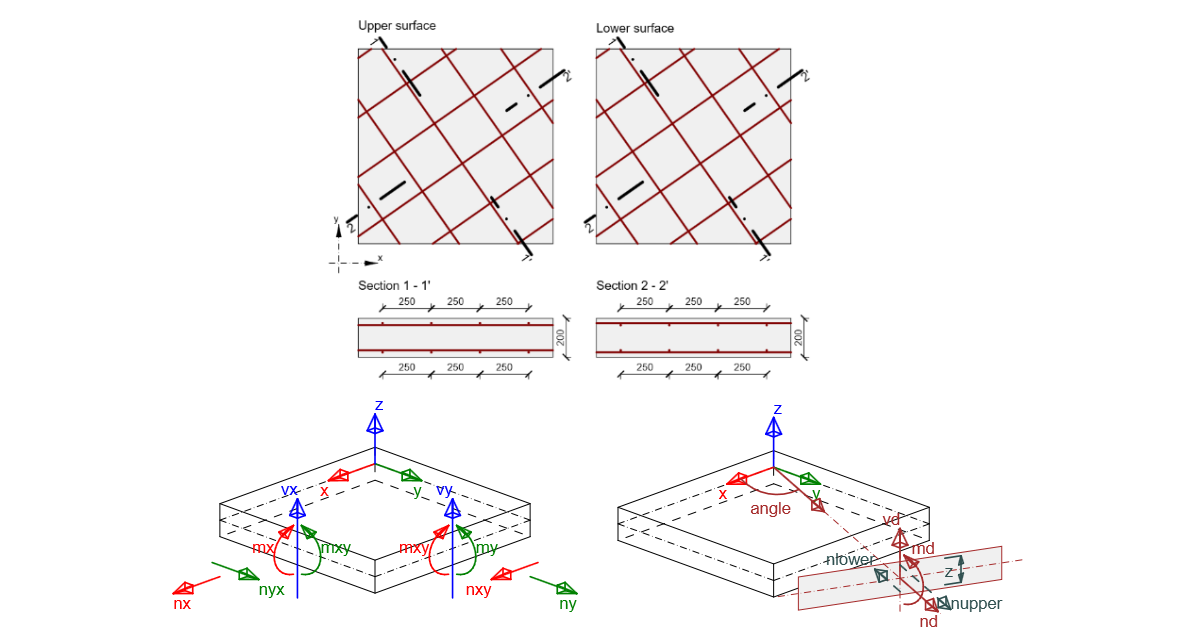# IDEA StatiCa RCS – Structural design of 2D concrete members

$$Design of reinforced concrete sections according to EN 1992-1-1 and EN 1992-2.Types of 2D membersReinforcement for 2D elementsInternal forcesCheck Types of 2D members SlabAccording to EN 1992-1-1, art. 5.3.1(4) a slab is a member, for which the minimum panel dimension is not less than 5 times the overall slab thickness. The slab is loaded only by bending moments and shear forces perpendicular to the centroidal plane of the slab. Detailing provisions check is performed according to EN 1992-1-1, art. 9.3.Shell as a slab – Shell-slabThe geometry is defined similarly to the slab geometry definition. Unlike the slab, the shell-slab can be loaded by bending and membrane actions. Detailing provisions are checked according to rules for slab (EN 1992-1-1, art. 9.3).WallAccording to EN 1992-1-1, art. 5.3.1(7) a wall is a member, for which the following principles are not fulfilled: the section depth does not exceed 4 times its width the height is at least 3 times the section depth The wall is loaded only by membrane action and detailing provisions are checked according to EN 1992-1-1, art. 9.6.Shell as wall – Shell-wallThe geometry is defined similarly to the wall geometry definition. Unlike the wall, the shell-wall can be loaded by bending and membrane actions. Detailing provisions are checked according to detailing provisions for wall (EN 1992-1-1, art. 9.6).Deep beamAccording to EN 1992-1-1, art. 5.3.1(3) a deep beam is a member for which the span is less than 3 times the overall section depth. The deep beam can be loaded as the wall only by membrane actions. Detailing provisions are checked according to EN 1992-1-1, art. 9.7. Reinforcement for 2D elements A shell element measuring 1m x 1m is defined for the check. Reinforcement is input into this shell element. Reinforcement per linear meter is taken into account for the check of the 2D member.Predefined reinforcement templates can be used to input the reinforcement to the upper and the lower edges. It is possible to input general reinforcement into the slab.The input of reinforcement using reinforcement templates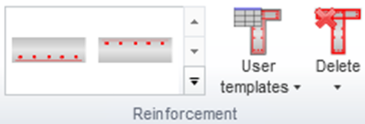IDEA RCS supplies two templates for the input of reinforcement into a 2D element. One template is for the input of reinforcement at the upper surface, the other one is for the input of reinforcement at the lower surface.Both templates allow input of orthogonal reinforcement at the surfaces of the 2D element. Both templates enable the rotation of reinforcement about the local x-axis of the 2D element.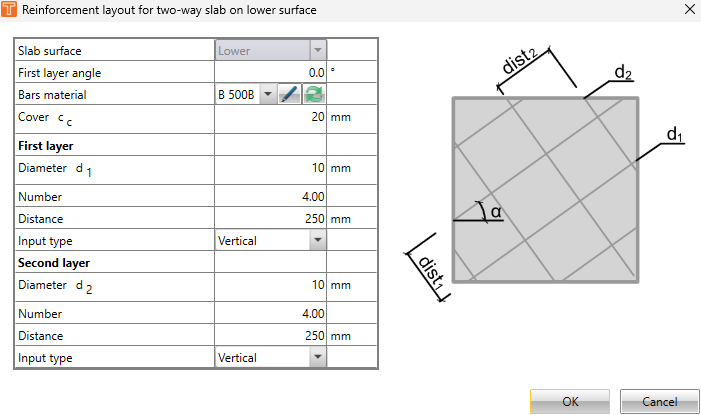$\textsf{\textit{\footnotesize{Dialog for the definition of 2D reinforcement}}}$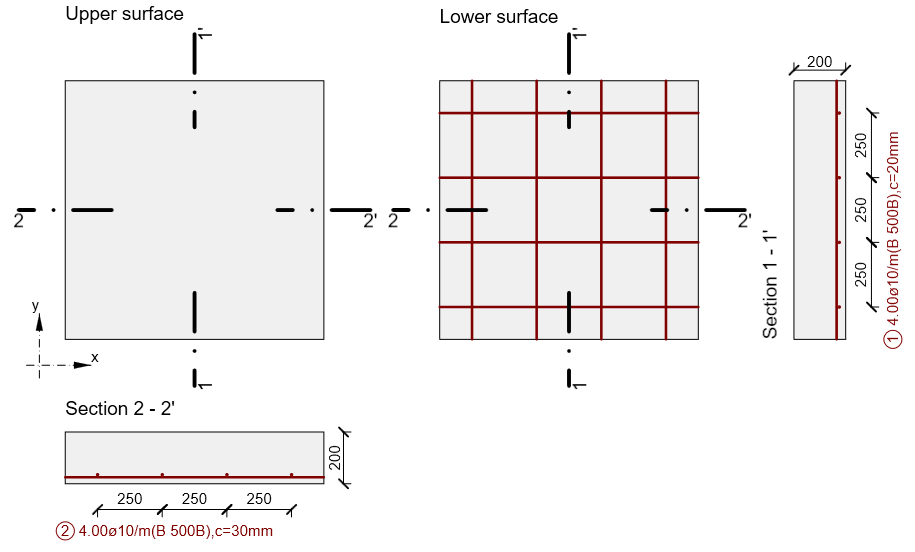$\textsf{\textit{\footnotesize{Schema of defined reinforcement at the lower surface of 2D element}}}$The input of general reinforcementEach reinforcement layer is defined in the section and in the plan.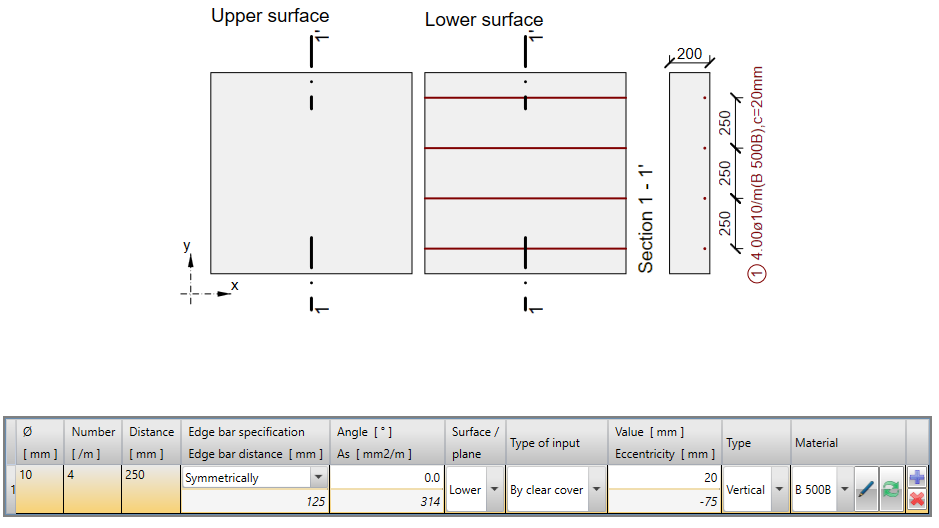$\textsf{\textit{\footnotesize{General input}}}$Type of reinforcementThe type of reinforcement bar has to be defined to be able to perform the check of detailing provisions. For 2D elements of type Slab and shell–slab – for checks according to EN 1992-1-1, art. 9.3.1.1 Main reinforcement Distribution reinforcement Wall, Shell-wall, and Deep beam – for check according to EN 1992-1-1, art 9.6.2 and 9.6.3 Horizontal reinforcement Vertical reinforcement Remark: The distribution reinforcement of slabs and shell-slabs is taken into account only for the check of detailing provisions, it is not used in other checks of the 2D elements. Internal forces for 2D sections The input of internal forcesThe input of internal forces of 2D members depends on the type of 2D element: Shell-slab – membrane forces (nx, ny and nxy), bending moments (mx, my and mxy) and shear forces (vx and vy) can be entered Shell- wall – membrane forces (nx, ny and nxy), bending moments (mx, my and mxy) and shear forces (vx and vy) can be entered Slab – only bending moments (mx, my and mxy) and shear forces (vx and vy) can be entered Wall – only membrane forces (nx, ny and nxy) can be entered Deep beam – only membrane forces (nx, ny and nxy) can be entered Description mx(y)Bending moment in the direction of x (y)-axis. A positive value causes tension at the lower surface of a 2D element. mxy(yx)Torsional moment about y (x)-axis acting on the edge parallel to the axis x (y). Positive value causes tensional shear stress at the lower surface of a 2D element. Because in each point of the 2D element theorem the equality of horizontal shear stresses is valid, torsional moments mxy = myx are equal in each point of 2D element too. Thus only value of mxy is entered in the program. nx(y)Normal force in direction of x (y)-axis. Positive value acts in direction of x(y) axis and causes tension in section. nxy(yx)Normal force acting in the centre plane in direction of y(x)-axis on edge parallel to x(y)-axis. Positive value acts in direction of x(y)-axis. Because in each point of the 2D element theorem the equality of horizontal shear stresses is valid, normal forces nxy = nyx are equal in each point of 2D element too. Thus only value of nxy is entered in the program. vx(y)Shear force acting perpendicular to centre plane on edge parallel to x(y)-axis. Positive value acts in direction of z-axis.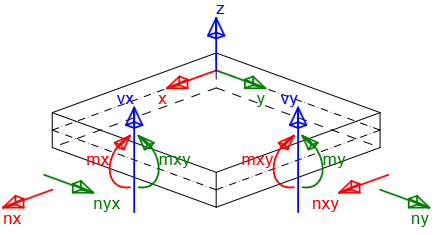$\textsf{\textit{\footnotesize{Sign convention of internal forces}}}$The following types of combinations have to be defined for checks: Ultimate limit state/Accidental – internal force components defined for this type of combinations are used for ULS checks of 2D elements: Capacity N-M-M Response N-M-M Interaction and the check of detailing provisions Characteristic – internal force components defined for this type of combination are used for check of stress limitation (SLS) Quasi-permanent – internal force components defined for this type of combination are used for check of crack width (SLS)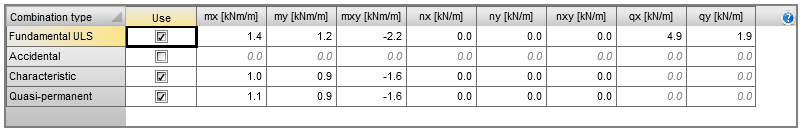Remark: Internal forces components vx and vy are not required to be entered for combination types Characteristic and Quasi-permanent, because these values are not used in checks. Determining the direction of the checkThe direction of the check has to be determined for the proper check of the 2D element. The direction of the check can be entered for each combination type separately, using the following two methods: User defined direction – the user defines the check direction as an angle relative to the x-axis in plane of the 2D element. This option is set as default for combination type ULS and the predefined value of the angle is 0 degrees. Checks are performed in the following directions: Defined direction Direction perpendicular to defined direction Direction of compression diagonal at the upper surface Direction of compression diagonal at the lower surface Direction of principal stresses – the check direction is calculated automatically as the direction of principal stresses at the upper and at the lower surface of the 2D element. This option is set as default for combination types Characteristic and Quasi-permanent. Checks are performed in the following directions: Direction of principal stresses at the lower surface Direction perpendicular to the direction of principal stresses at the lower surface Direction of compression diagonal at the lower surface Direction of principal stresses at the upper surface Direction perpendicular to the direction of principal stresses at the upper surface Direction of compression diagonal at the upper surface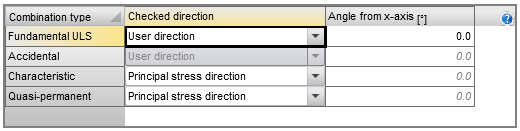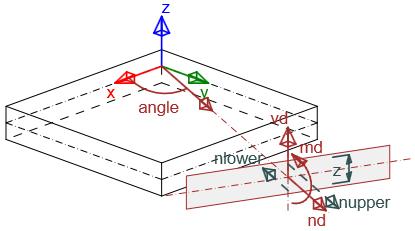$\textsf{\textit{\footnotesize{Recalculated internal forces in input direction by theory of Baumann}}}$Analysis of check direction for ultimate limit stateAnalysis 1For a 2D element loaded only by bending moments (mx = 20 kNm/m, my = 10 kNm/m, mxy = 5 kNm/m ) with the angle of reinforcement and angle of check direction changed for the ultimate limit state - the results are displayed in the following graph: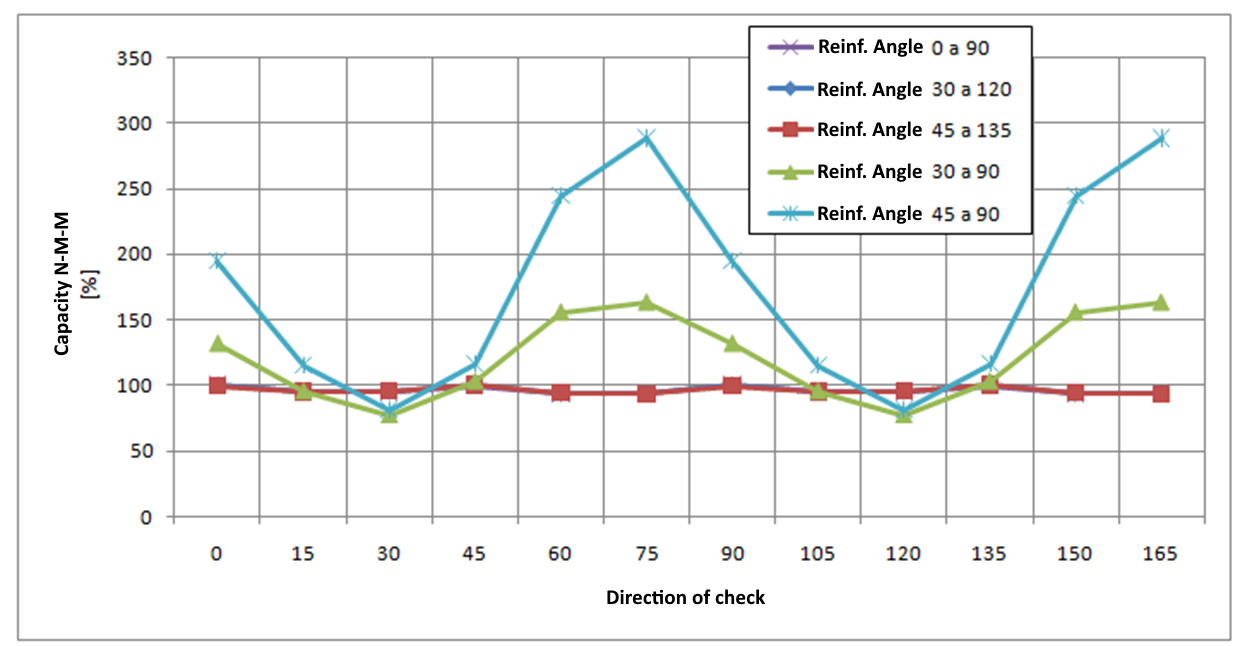The analysis implies: If reinforcement bars are perpendicular to each other, check results are similar for different check direction angles, they are not dependent on the defined reinforcement angle and the maximum value of the check is found for angles 0, 45, and 90 degrees. Thus this check can be performed for a predefined direction of a check angle of 0 degrees. If reinforcement bars are not perpendicular to each other, the results of the checks differ significantly and the maximum check value is achieved approximately in the direction corresponding with the direction of average reinforcement. Thus it is recommended to change the predefined check direction or to perform checks in more directions in cases when reinforcement bars are not perpendicular to each other. Analysis 2For the orthogonal reinforcement, the values of bending moments and the angle have been changed for the ULS code-check. Results are represented in the graph: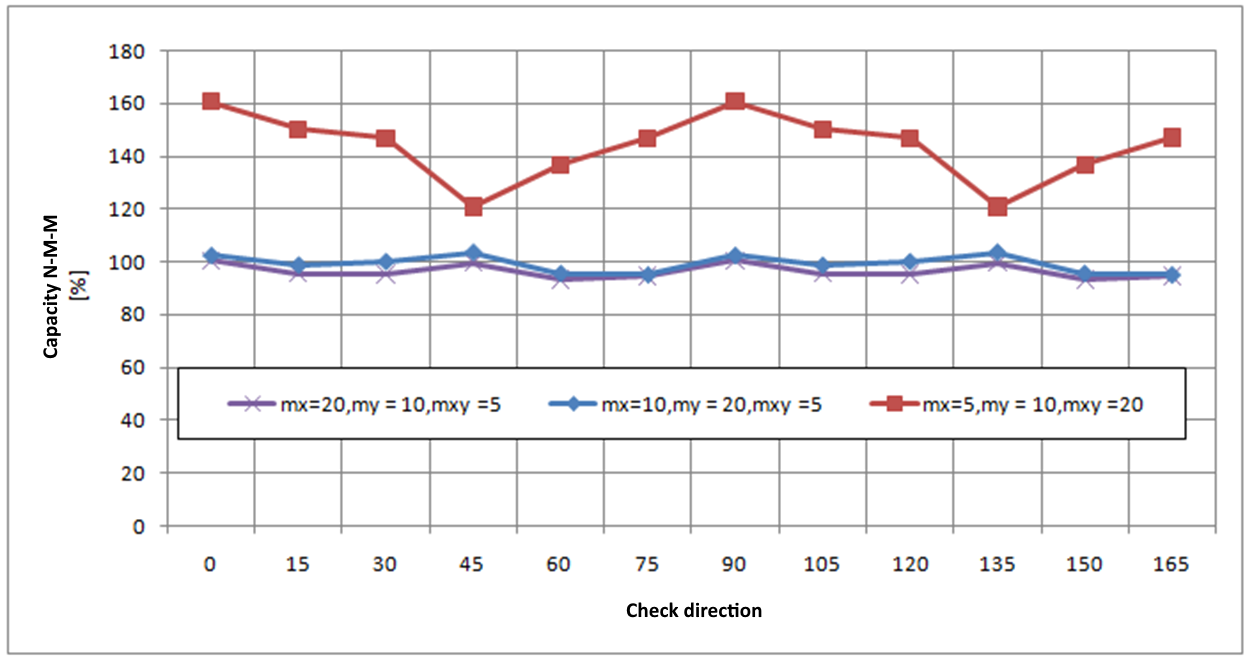The analysis implies that even for different values of bending moments the maximum value of the ultimate limit state check is found for check directions 0, 45, and 90 degrees. Thus the check can be performed for a predefined check angle of 0 degrees. A similar conclusion is valid for 2D elements loaded by normal force only or loaded by normal force combined with bending moments.Recalculation of internal forces to directions of checkThe defined internal forces are recalculated to the check directions using the Baumann transformation formula, described in Baumann, Th. : "Zur Frage der Netzbewehrung von Flächentragwerken". In : Der Bauingenieur 47 (1972), Berlin 1975. The calculation procedure is as follows: Calculation of normal forces at both surfaces of the 2D element Calculation of principal forces at both surfaces of the 2D element Calculation of recalculated forces for each surface to the defined direction of check Calculation of recalculated forces for each surface to the center Recalculation of shear forces to the defined direction of check Calculation of normal forces at both surfaces of the 2D elementDefined internal forces are recalculated to both surfaces using the following formulas:${{n}_{x,low\left( upp \right)}}=\frac{{{n}_{x}}}{2}+\left( - \right)\frac{{{m}_{x}}}{z}$${{n}_{y,low\left( upp \right)}}=\frac{{{n}_{y}}}{2}+\left( - \right)\frac{{{m}_{y}}}{z}$$~~~~~{{n}_{xy,low\left( upp \right)}}=\frac{{{n}_{xy}}}{2}+\left( - \right)\frac{{{m}_{xy}}}{z}$The lever arm of internal forces (z) has to be determined for the recalculation of internal forces. The lever arm of internal forces is determined from the method of limit strain at loading by the principal bending moment in directions of the principal moments m1 at both surfaces. If the principal moments are equal to zero or if the equilibrium is not found in the direction of principal moments, the lever arm of internal forces is determined according to the formula:$z=x\cdot d$ Description xThe coefficient for calculation of the internal forces arm is defined in the National code setup. dThe effective height of the cross-section calculated separately for the upper and the lower surfaces of the 2D element. For the lower surface, it is a distance from the centroid of reinforcement bars at the lower surface to the upper edge of the cross-section. For the upper surface, it is a distance from the centroid of reinforcement bars at the upper surface to the lower edge of the cross-section.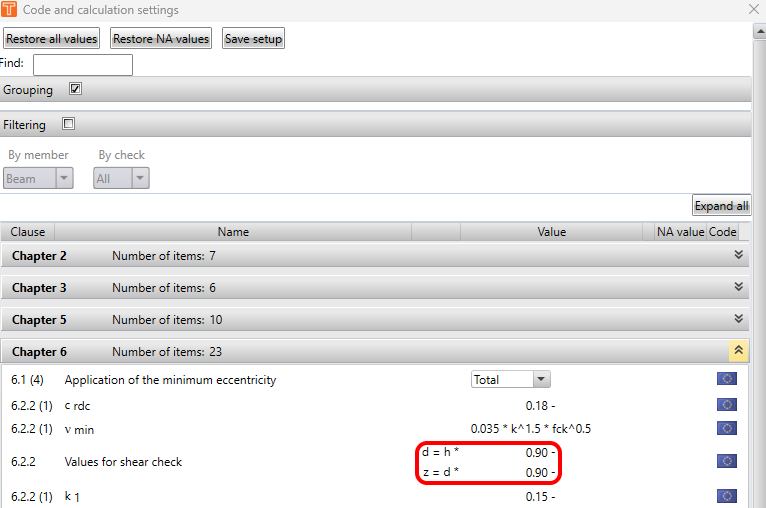Remark: The arm of internal forces can be verified in the Response N-M-M check. Only the bending moments have to be entered and the check direction has to correspond with the direction of the principal moment. In the following diagram, a verification of the internal forces lever arm is displayed for bending moments mx = 20 kNm/m, my = 10 kNm/m, mxy = 5 kNm/m. The direction of principal moments has been calculated as αm1 = 22.5 degrees and the response of cross-section was calculated to determine the internal forces lever arm.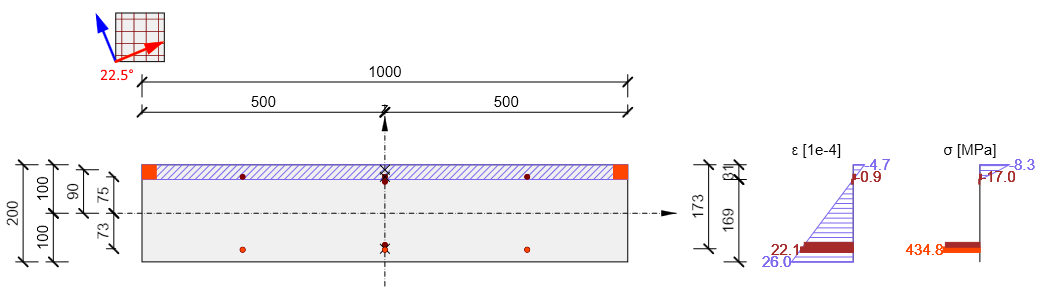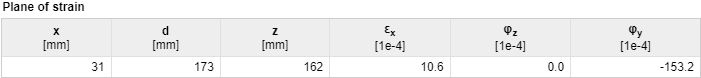Remark: Internal force lever arms for recalculation of internal forces in the direction of the check and internal force lever arms for checks can be different, because the internal force lever arm for recalculation is determined on a cross-section loaded by principal moments in the direction of principal moments, and the internal force lever arm for the check is determined on a cross-section loaded by bending moments and normal forces in the direction of the check. Values of internal force lever arms for all combination types are displayed in the table Recalculated forces in the navigator Internal forces in section.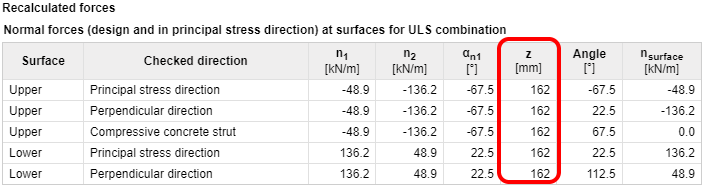Calculation of internal forces at both surfacesPrincipal forces at both surfaces of the 2D element are calculated using the formula:${{n}_{1,bot\left( top \right)}}=\frac{{{n}_{x,low\left( upp \right)+}}{{n}_{y,low\left( upp \right)}}}{2}+\frac{1}{2}\sqrt{{{\left( {{n}_{x,low\left( upp \right)-}}{{n}_{y,low\left( upp \right)}} \right)}^{2}}+4\cdot {{n}_{xy,low\left( upp \right)}}}$${{n}_{2,bot\left( top \right)}}=\frac{{{n}_{x,low\left( upp \right)+}}{{n}_{y,low\left( upp \right)}}}{2}-\frac{1}{2}\sqrt{{{\left( {{n}_{x,low\left( upp \right)-}}{{n}_{y,low\left( upp \right)}} \right)}^{2}}+4\cdot {{n}_{xy,low\left( upp \right)}}}$And the direction of principal forces is calculated using the formula:${{\alpha }_{n1,low\left( upp \right)}}=0,5\cdot {{\tan }^{-1}}\left( \frac{2\cdot {{n}_{xy,low\left( upp \right)}}}{{{n}_{x,low\left( upp \right)}}-{{n}_{y,low\left( upp \right)}}} \right)$ Remark: Principal forces and the direction of principal forces for both surfaces of the 2D element are displayed for all combination types in the table Recalculated forces in the navigator Internal forces in section.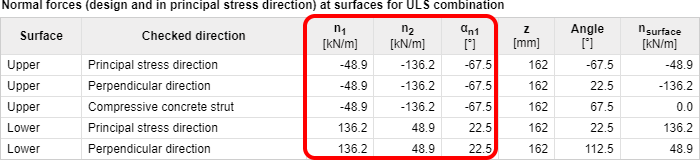Calculation of recalculated internal forces at surfaces to the defined check directionRecalculation of principal forces to the check directions is performed separately for each surface using the Baumann transformation formula:${{n}_{surface,i,low\left( upp \right)}}=\frac{{{n}_{1,low\left( upp \right)}}\cdot \sin \left( {{\alpha }_{j,low\left( upp \right)}} \right)\cdot \sin \left( {{\alpha }_{k,low\left( upp \right)}} \right)+{{n}_{2,low\left( upp \right)}}\cdot \cos \left( {{\alpha }_{j,low\left( upp \right)}} \right)\cdot \cos \left( {{\alpha }_{k,low\left( upp \right)}} \right)}{\sin \left( {{\alpha }_{j,low\left( upp \right)}}-{{\alpha }_{i,low\left( upp \right)}} \right)\cdot \sin \left( {{\alpha }_{k,low\left( upp \right)}}-{{\alpha }_{i,low\left( upp \right)}} \right)}$ Description i, j, k, iIndex of check direction (internal forces recalculation direction) i, j, k, i = 1, 2, 3, 1 . E. G. For lower surface and calculation of force in j-direction (angle α2) the formula is: ${{n}_{surface,2,low}}=\frac{{{n}_{1,low}}\cdot \sin {{\alpha }_{3,low}}\cdot \sin {{\alpha }_{1,low}}+{{n}_{2,low}}\cdot \cos {{\alpha }_{3,low}}\cdot \cos {{\alpha }_{1,low}}}{\sin \left( {{\alpha }_{3,low}}-{{\alpha }_{2,low}} \right)\cdot \sin \left( {{\alpha }_{1,low}}-{{\alpha }_{2,low}} \right)}$ ${{\alpha }_{i,j,k,low\left( upp \right)}}$The angle between the defined check direction or the direction of compressive strut and the direction of principal forces at the lower or upper surface of the 2D element. Defined check direction α1, low(upp) = α1 – α low(upp) Dir. perpendicular to the defined direction α2, low(upp) = α2 – α low(upp) The direction of check for compressive strut α3, low(upp) = α3 – α low(upp) α1Defined check direction for the particular combination α2The direction perpendicular to the defined direction, α2 = α1 + 90 degrees α3Check the direction in the direction of the compressive strut in the plane of the 2D element. This direction is optimized to minimize the force in this direction. Remark: If the check direction is identical to the Principal stresses direction, the forces in the compressive strut are zero, thus this direction is neglected in the check The direction of the compressive strut for all states of stress except the hyperbolic state of stress (n1,low(upp) > 0 and n1,low(upp) < 0) can be calculated according to the formula: α3 = 0,5(α1 + α2) Recalculated internal forces for both surfaces of 2D element and all check directions including the direction of the compressive strut are displayed in the table Recalculated forces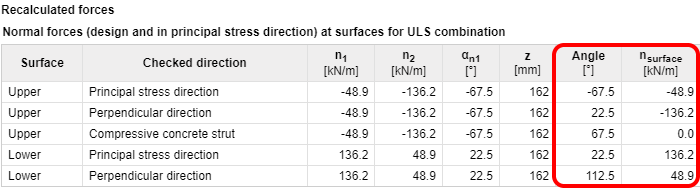Transformation of recalculated internal forces to the centroid of the cross-sectionFor the check of the 2D element, the surface forces in a particular direction have to be recalculated to the centroid of the cross-section. The result is normal force nd,i and bending moment md,I acting in the centroid of the 2D element cross-section. md,i = nlower,i·zs,low + nupper,i·zs,upp nd,i = nlower,i + nupper,i Description nlower,iRecalculated surface forces at lower surface in the ith check direction, when nlower,i = nsurface,low,i. nupper,iRecalculated internal forces at upper surface in the ith check direction, when nupper,i = nsurface,upp,i. zs,low (upp)Distance of centroid of compressed concrete or centroid of reinforcement at the lower (upper) surface, when z = zs,low + zs,upp Remark: If the directions of compressive struts at the lower and the upper surface are different, for the recalculation of forces to the centroid it is necessary to calculate virtual forces at the bottom surface in the direction of the compressive strut at the upper surface and vice versa.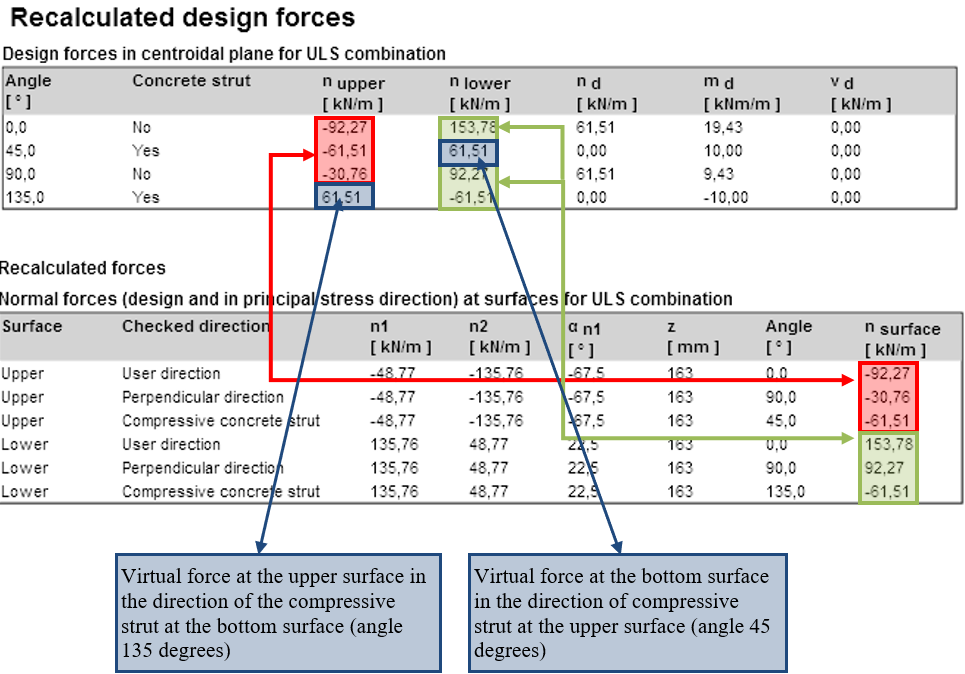$\textsf{\textit{\footnotesize{Recalculated design forces}}}$Recalculation of shear forces to the defined direction of checkShear forces are recalculated to the direction of the check using the formula:${{v}_{d,i}}={{v}_{x}}\cdot \cos ({{\alpha }_{i}})+{{v}_{y}}\cdot \sin ({{\alpha }_{i}})$and the maximal shear force is:${{v}_{d,max~}}=\sqrt{{{v}_{x}}^{2}+{{v}_{y}}^{2}}$and it acts in the direction$\beta ={{\tan }^{-1}}\left( \frac{{{v}_{y}}}{{{v}_{x}}} \right)$ Description αiCheck the angle in the ith direction Remark: When checking a 2D element with relatively large shear forces it is suitable to check the 2D element in the direction of maximal shear force, which means that the defined direction check corresponds to angle β Comparison of internal forces recalculation using various methodsRecalculation of forces acc. to EN 1992-1-1The method described in EN 1992-1-1 is used in several programs and in practice to calculate design internal forces. EN 1992-1-1 takes into account only perpendicular reinforcement directions. Calculation of dimensioning forces with the influence of torsional moment is described in the following flowchart, where my³ mx. A similar diagram can be created for moments my < mx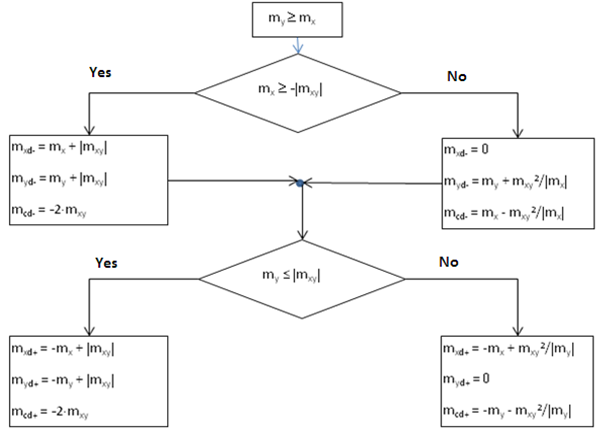Description mxd+, mxd-Dimensioning bending moment in the x-axis direction for design and check of reinforcement at the lower (-) or the upper (+) surface myd+ myd- Dimensioning bending moment in the y-axis direction for design and check of reinforcement at the lower (-) or the upper (+) surface mcd+, mcd-Dimensioning bending moment in the compressive concrete strut at the lower (-) or the upper (+) surface, which has to be carried by concrete Values of recalculated dimensioning forces for the type of member = Slab, calculated using the method described in EN, are displayed in the following table: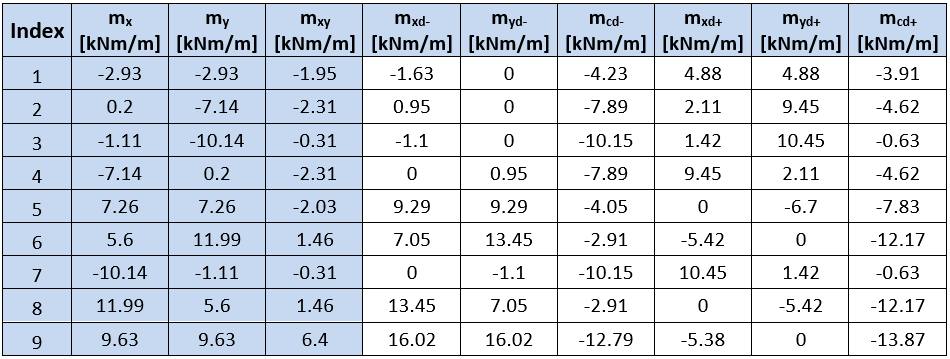In IDEA StatiCa RCS values of moments at the upper and lower surface are not displayed, but values of normal forces at both surfaces and values of moments recalculated to the centroid of the cross-section.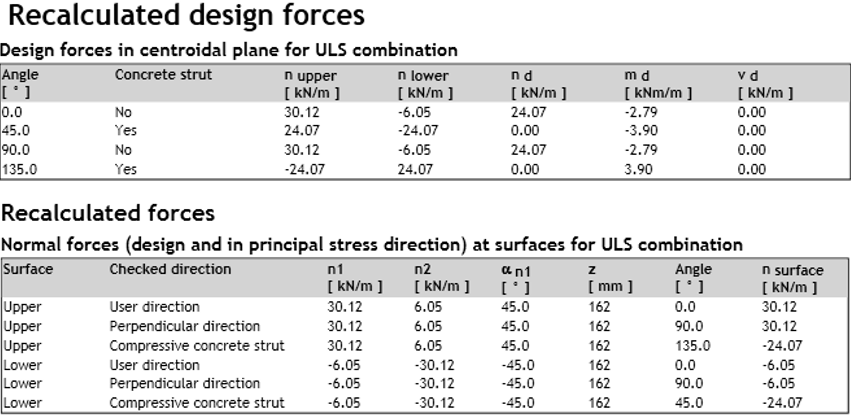Moments at the lower and upper surfaces can be calculated using surface forces, which are displayed in the numerical output, using the formula:${{m}_{surface,i,dlow\left( upp \right)}}={{n}_{surface,i,low\left( upp \right)}}\cdot z$Values of surface forces and recalculated moments are displayed in the following tables: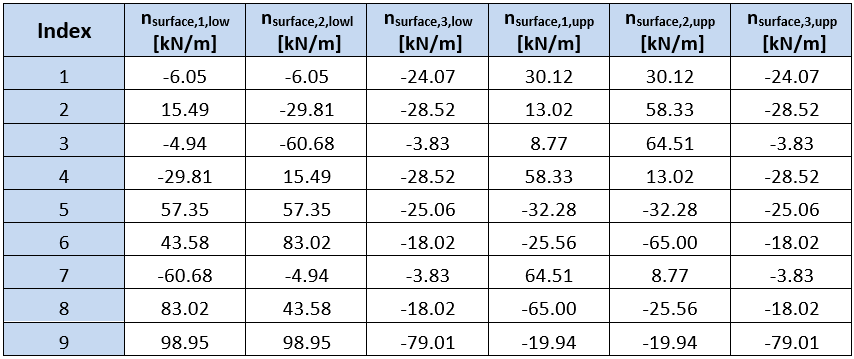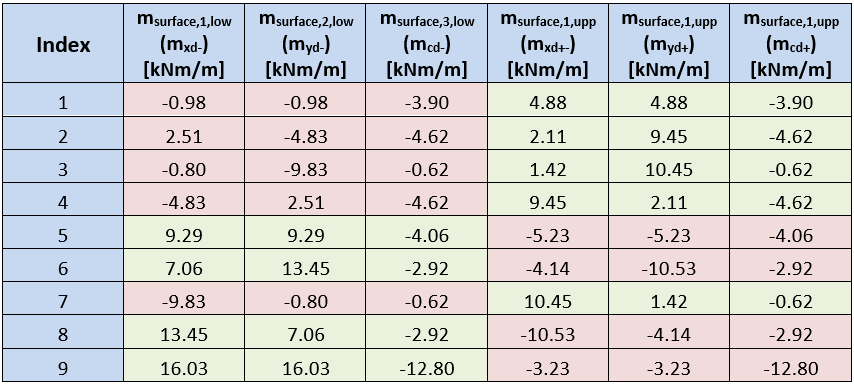The tables show, that moments at slab surfaces calculated in IDEA Concrete and calculated according to the method described in EN, correspond only at one surface. This difference is caused by different optimization of the concrete strut. The method used in IDEA StatiCa RCS searches for the angle of the compressive strut at the minimal force in the strut. The method described in EN searches for a minimal sum of negative forces from all directions.Comparison of internal forces calculation with programs RFEM and SCIA EngineerTo compare the results of recalculated internal forces in programs IDEA Concrete, RFEM, and SCIA Engineer (SEN) a simple model of slab of dimensions 6 m x 4 m and thickness 200 mm was prepared. The slab is supported with line support at the edges and loaded with a uniform load of 10 kN/m2.To simplify the presentation only values of recalculated internal forces in one longitudinal section are displayed. The section distance from the slab edge is 1.5m. The internal forces calculated in the program RFEM were used as input values for IDEA Concrete.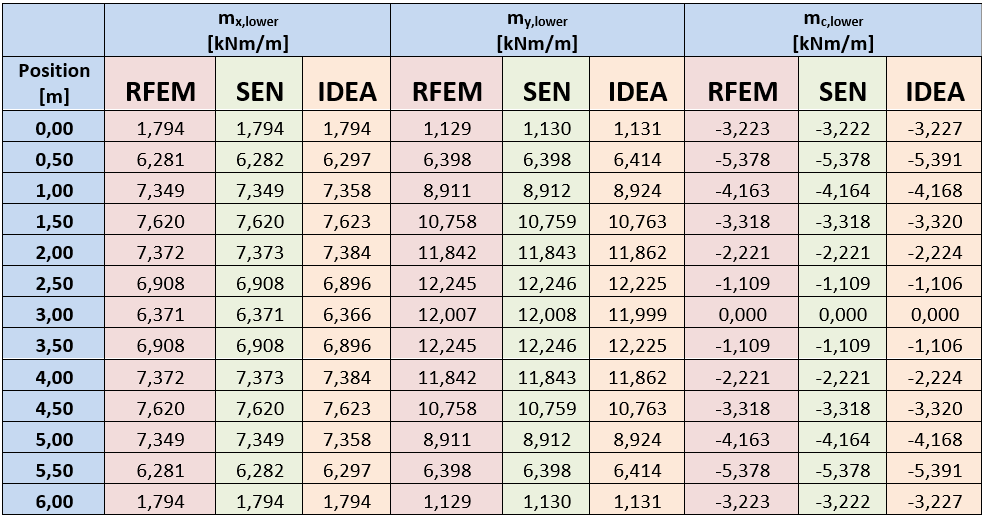The table shows good compliance of forces calculated in particular programs. Check As described in Internal forces in the chapter Transformation of recalculated internal forces to the centroid of cross-section, surface dimensioning forces are transformed to the centroid of a 2D element cross-section. The result of this transformation is a bending moment and a normal force, acting in the centroid of a rectangular cross-section, in which the edge length is 1 m and height corresponds to the thickness of the slab.The checks of the 2D element are performed in all defined directions at once. The program automatically converts the reinforcement to the check direction using the formula:${{A}_{Si,\alpha }}={{A}_{S}}\cdot {{\cos }^{2}}({{\alpha }_{i}})$ Description Asi,aThe area of the ith reinforcement layer recalculated to the direction a AsThe area of the ith reinforcement layer of the 2D element αiThe angle between ith reinforcement layer and the check direction Remark: Distribution reinforcement in 2D elements of type slab and shell-slab is taken into account only in the detailing provision check, it is not used in other 2D element checks. Results of checks in defined directionsAll enabled checks are performed in all required directions automatically. The presentation of results is similar to the presentation of 1D element results. The presentation for 2D elements enables to set the direction to be presented. Results for 2D elements are presented in check directions. All directions, in which the checks were calculated, are drawn in graphical presentation.The arrows in the picture represent check directions, where the orange is the direction of the maximum check value and the red is the current check direction. To change the current direction click the arrow or click the appropriate button in the ribbon.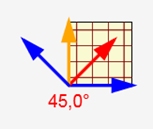Remark: After the calculation finishes, the check directions in all checks are set to the direction of maximum cross-section utilization. Results in particular checks are presented in the current direction. The angle of the check is displayed above the table with the check summary.The results in the extreme direction are printed in the report.Ultimate limit stateThe principles of ULS checks are described in the theoretical background manual for 1D elements. Only the differences for 2D elements are described in the following chapters.Capacity checkThe capacity check does not differ from the 1D elements checks. The load acts only in one plane, thus the check type is N + M.Response checkThe response checks for particular check directions use the same algorithms as checks of 1D elements.Interaction checkUnlike for 1D elements, the interaction check is performed only to evaluate the exploitation V + M, the interaction of shear and bending moment. Values VRd,c and VRd,max can be verified in the summary table of the interaction check.Capacity check comparison between IDEA Concrete, RFEM, and SCIA EngineerTo compare the capacity check results with RFEM and SCIA Engineer the same data as described in Internal forces in the chapter Comparison of internal forces calculation with programs RFEM and SCIA Engineer were used. The comparison was done in two points of the slab.Because programs RFEM and SEN do not check the real reinforcement in the slab, but only design the necessary reinforcement area, two methods were used to compare the calculation. The first one compares the exploitation of cross-section for required reinforcement designed in RFEM and SEN, assuming that the cross-section is exploited to 100% just when using the calculated required area of reinforcement.The exploitation of cross-section reinforced in IDEA concrete can be expressed relatively then.Relative exploitation = As, req / As, RCS × 100 [%] Description As, reqRequired reinforcement area calculated in RFEM or SEN As, RCSArea of reinforcement in IDEA Concrete 100 [%]Percentage The cross-section in IDEA Concrete was reinforced at the lower surface using reinforcement d=10 mm in 200 mm distances in both directions, the area of reinforcement in both directions is 314 mm2.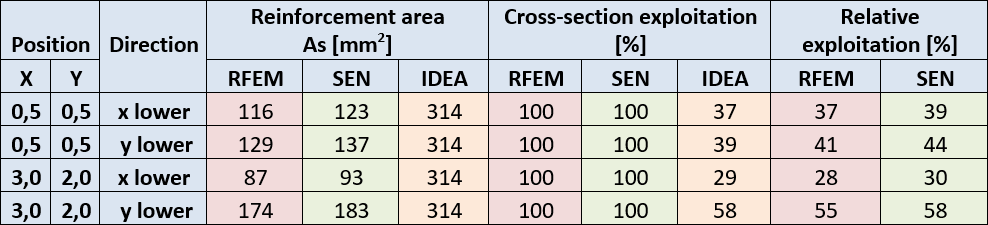The table shows good compliance with exploitation for all programs.Reinforcement with approximately same area was defined in IDEA Concrete as the calculated required reinforcement in RFEM and SEN for the second method. Afterwards, the exploitation of the cross-section was compared. Results are displayed in the following table: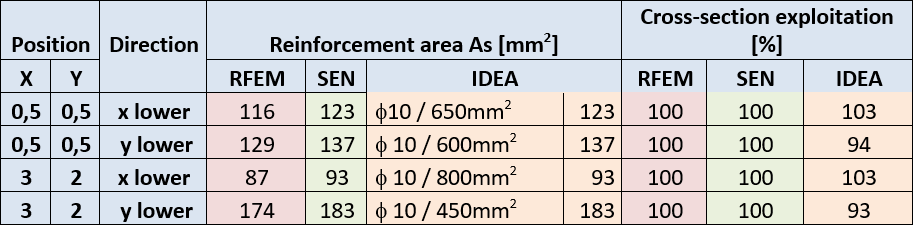The good compliance of results is here too.Serviceability limit stateStress limitationCheck of stress limitation does not differ from checks for 1D elements.Crack width checkIn advance, 1D elements check the direction of the crack that can be drawn for 2D elements.Detailing provisionsDetailing provisions check of 2D elements can be split into two basic groups: Reinforcement percentage check Bar distances check The detailing provisions check depends on the type of 2D element too. The separate checks for the main and for distribution reinforcement are performed for shell-slab and slab elements. The vertical and horizontal reinforcement is distinguished for wall elements.The reinforcement percentage check is performed in the direction of principal stresses. The reinforcement defined in the cutting of 2D element (except the distribution reinforcement) is transformed to the principal stresses directions.The bars distance check is performed perpendicular to the direction of the defined reinforcement. This check is performed for all defined reinforcement layers and the limit values depend on the type of checked element and on the type of defined reinforcement.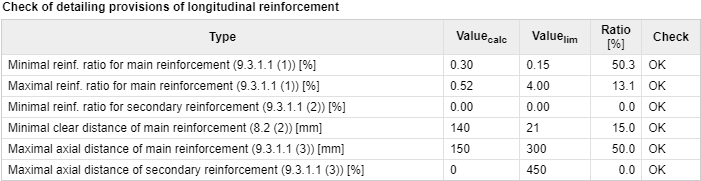Try IDEA StatiCa for free Start your trial today and enjoy 14-days of full access and services free of charge. Start free trial$$